# Neural Network Models in R

In this tutorial, you will learn how to create a Neural Network model in R.

Neural Network (or Artificial Neural Network) has the ability to learn by examples. ANN is an information processing model inspired by the biological neuron system. It is composed of a large number of highly interconnected processing elements known as the neuron to solve problems. It follows the non-linear path and process information in parallel throughout the nodes. A neural network is a complex adaptive system. Adaptive means it has the ability to change its internal structure by adjusting weights of inputs. (Source)

The neural network was designed to solve problems which are easy for humans and difficult for machines such as identifying pictures of cats and dogs, identifying numbered pictures. These problems are often referred to as pattern recognition. Its application ranges from optical character recognition to object detection.

In this tutorial, you are going to cover the following topics:

• Introduction to neural network
• Forward Propagation and Back Propagation
• Activation Function
• Implementation of the neural network in R
• Use-cases of NN
• Pros and Cons
• Conclusion

## Introduction to Neural Network

In 1943, Warren McCulloch and Walter Pitts developed the first mathematical model of a neuron. In their research paper "A logical calculus of the ideas immanent in nervous activity”, they described the simple mathematical model for a neuron, which represents a single cell of the neural system that takes inputs, processes those inputs, and returns an output. This model is known as the McCulloch-Pitts neural model. (Source)

NN is algorithms are inspired by the human brain to performs a particular task or functions. NN perform computations through a process by learning. The neural network is a set of connected input/output units in which each connection has a weight associated with it. In the learning phase, the network learns by adjusting the weights to predict the correct class label of the given inputs.

The human brain consists of billions of neural cells that process information. Each neural cell considered a simple processing system. The Interconnected web of neurons known as biological neural network transmits information through electrical signals. This parallel interactive system makes the brain to think and process information. Dendrites of a neuron receive input signals from another neuron and respond output based on those inputs to an axon of some other neuron. Based on those inputs, fire an output signal via an axon. (Source)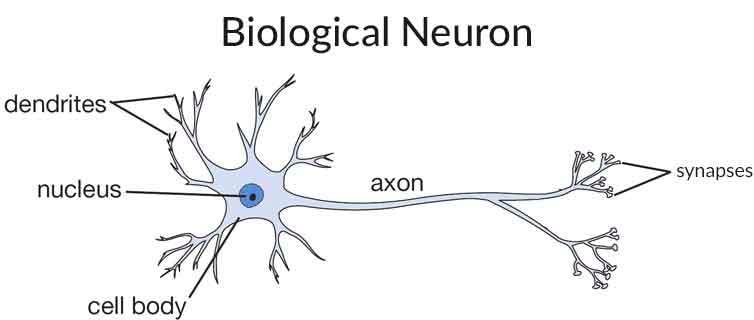Image Source

Dendrites receive signals from other neurons. Cell body sums all the inputs signals to generate output. Axon through output When the sum reaches to a threshold. Synapses is a point of interaction neurons. It transmits electrical or chemical signals to another neuron. Synapse is derived from the Greek word which means conjunction.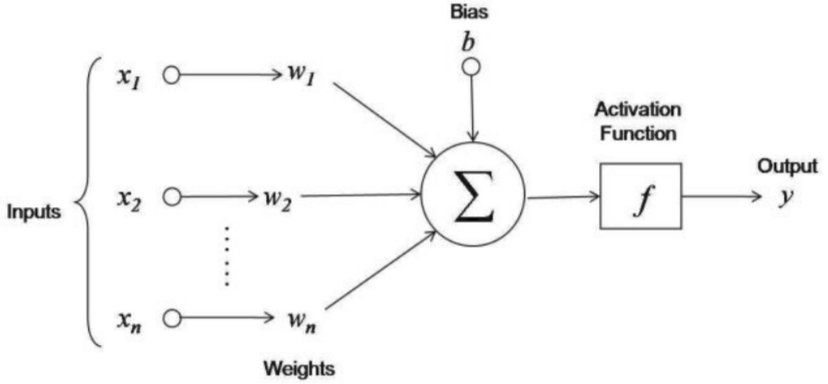Image Source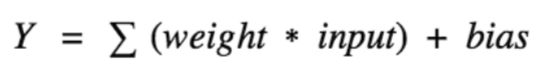Here, x1,x2....xn are input variables. w1,w2....wn are weights of respective inputs. b is the bias, which is summed with the weighted inputs to form the net inputs. Bias and weights are both adjustable parameters of the neuron. Parameters are adjusted using some learning rules. The output of a neuron can range from -inf to +inf. The neuron doesn’t know the boundary. So we need a mapping mechanism between the input and output of the neuron. This mechanism of mapping inputs to output is known as Activation Function.

## Feedforward and Feedback Artificial Neural Networks

There are two main types of artificial neural networks: Feedforward and feedback artificial neural networks. Feedforward neural network is a network which is not recursive. Neurons in this layer were only connected to neurons in the next layer, and they are don't form a cycle. In Feedforward signals travel in only one direction towards the output layer. (Source)

Feedback neural networks contain cycles. Signals travel in both directions by introducing loops in the network. The feedback cycles can cause the network's behavior change over time based on its input. Feedback neural network also known as recurrent neural networks. (Source)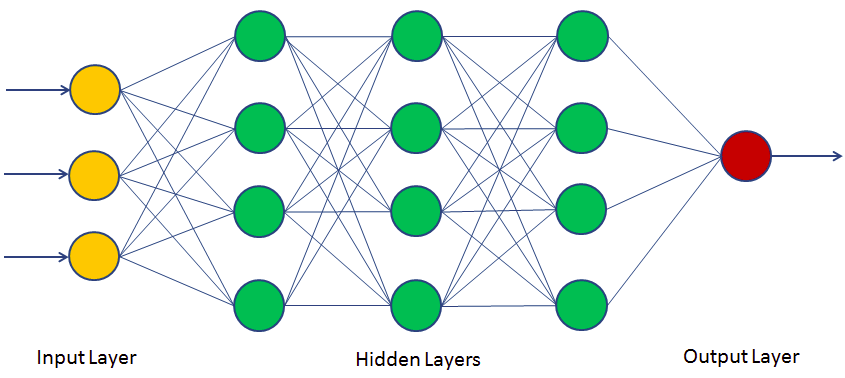## Activation Functions

Activation function defines the output of a neuron in terms of a local induced field. Activation functions are a single line of code that gives the neural nets non-linearity and expressiveness. There are many activation functions. Some of them are as follows (Source):

• Identity function is a function that maps input to the same output value. It is a linear operator in vector space. Also, known straight line function where activation is proportional to the input.
• In Binary Step Function, if the value of Y is above a certain value known as the threshold, the output is True(or activated), and if it’s less than the threshold, then the output is false (or not activated). It is very useful in the classifier.
• Sigmoid Function called S-shaped functions. Logistic and hyperbolic tangent functions are commonly used sigmoid functions. There are two types of sigmoid functions.
• Binary Sigmoid Function is a logistic function where the output values are either binary or vary from 0 to 1.
• Bipolar Sigmoid Function is a logistic function where the output value varies from -1 to 1. Also known as Hyperbolic Tangent Function or tanh.
• Ramp Function: The name of the ramp function is derived from the appearance of its graph. It maps negative inputs to 0 and positive inputs to the same output.
• ReLu stands for the rectified linear unit (ReLU). It is the most used activation function in the world. It output 0 for negative values of x.## Implementation of a Neural Network in R

### Install required package

Let's first install the neuralnet library:

``````# install package
install.packages("neuralnet")
``````
``````Updating HTML index of packages in '.Library'
Making 'packages.html' ... done
``````

### Create training dataset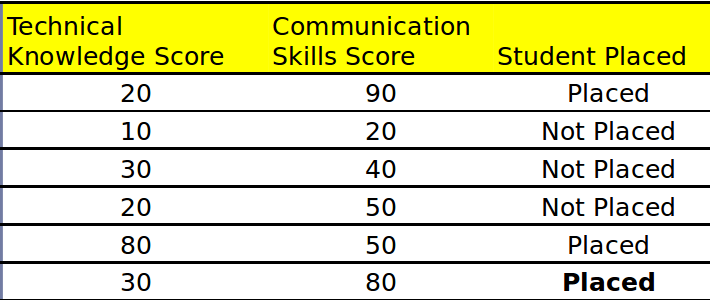Let's create your own dataset. Here you need two kinds of attributes or columns in your data: Feature and label. In the table shown above, you can see the technical knowledge, communication skills score and placement status of the student. So the first two columns(Technical Knowledge Score and Communication Skills Score) are features and third column(Student Placed) is the binary label.

``````# creating training data set
TKS=c(20,10,30,20,80,30)
CSS=c(90,20,40,50,50,80)
Placed=c(1,0,0,0,1,1)
# Here, you will combine multiple columns or features into a single set of data
df=data.frame(TKS,CSS,Placed)
``````

Let's build a NN classifier model using the neuralnet library.

First, import the neuralnet library and create NN classifier model by passing argument set of label and features, dataset, number of neurons in hidden layers, and error calculation.

``````# load library
require(neuralnet)

# fit neural network
nn=neuralnet(Placed~TKS+CSS,data=df, hidden=3,act.fct = "logistic",
linear.output = FALSE)
``````

Here, - Placed~TKS+CSS, Placed is label annd TKS and CSS are features. - df is dataframe, - hidden=3: represents single layer with 3 neurons respectively. - act.fct = "logistic" used for smoothing the result. - linear.ouput=FALSE: set FALSE for apply act.fct otherwise TRUE

### Plotting Neural Network

Let's plot your neural net model.

``````# plot neural network
plot(nn)
``````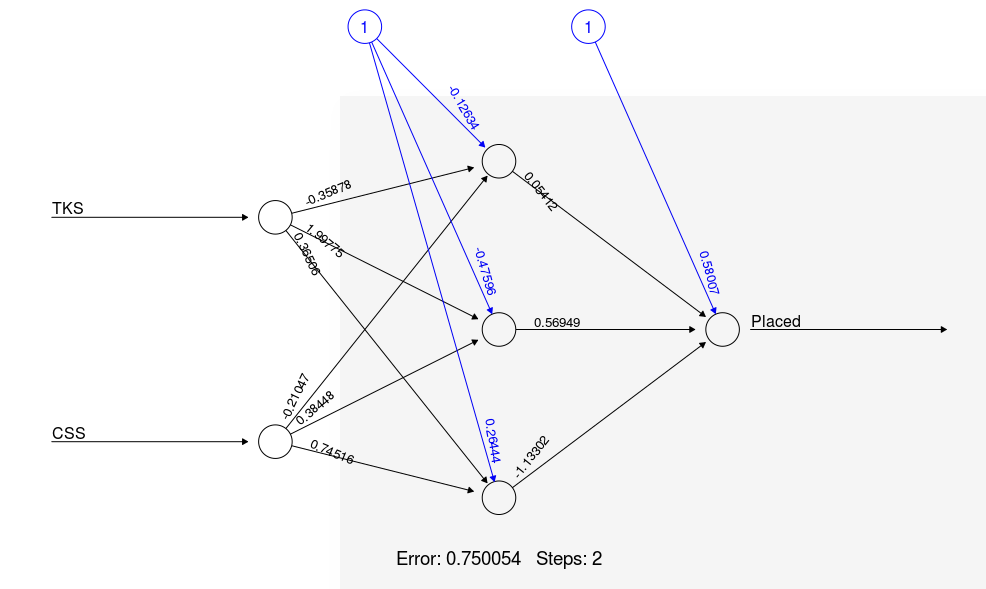### Create test dataset

Create test dataset using two features Technical Knowledge Score and Communication Skills Score

``````# creating test set
TKS=c(30,40,85)
CSS=c(85,50,40)
test=data.frame(TKS,CSS)
``````

### Predict the results for the test set

Predict the probability score for the test data using the compute function.

``````## Prediction using neural network
Predict=compute(nn,test)
Predict\$net.result
``````
 0.99282 0.333554 0.977515

Now, Convert probabilities into binary classes.

``````# Converting probabilities into binary classes setting threshold level 0.5
prob <- Predict\$net.result
pred <- ifelse(prob>0.5, 1, 0)
pred
``````
 1 0 1

Predicted results are 1,0, and 1.

## Pros and Cons

Neural networks are more flexible and can be used with both regression and classification problems. Neural networks are good for the nonlinear dataset with a large number of inputs such as images. Neural networks can work with any number of inputs and layers. Neural networks have the numerical strength that can perform jobs in parallel.

There are more alternative algorithms such as SVM, Decision Tree and Regression are available that are simple, fast, easy to train, and provide better performance. Neural networks are much more of the black box, require more time for development and more computation power. Neural Networks requires more data than other Machine Learning algorithms. NNs can be used only with numerical inputs and non-missing value datasets. A well-known neural network researcher said "A neural network is the second best way to solve any problem. The best way is to actually understand the problem,"

## Use-cases of NN

NN's wonderful properties offer many applications such as:

• Pattern Recognition: neural networks are very suitable for pattern recognition problems such as facial recognition, object detection, fingerprint recognition, etc.
• Anomaly Detection: neural networks are good at pattern detection, and they can easily detect the unusual patterns that don’t fit in the general patterns.
• Time Series Prediction: Neural networks can be used to predict time series problems such as stock price, weather forecasting.
• Natural Language Processing: Neural networks offer a wide range of applications in Natural Language Processing tasks such as text classification, Named Entity Recognition (NER), Part-of-Speech Tagging, Speech Recognition, and Spell Checking.

## Conclusion

Congratulations, you have made it to the end of this tutorial!

In this tutorial, you have covered a lot of details about the Neural Network. You have learned what Neural Network, Forward Propagation, and Back Propagation are, along with Activation Functions, Implementation of the neural network in R, Use-cases of NN, and finally Pros, and Cons of NN.

Hopefully, you can now utilize Neural Network concept to analyze your own datasets. Thanks for reading this tutorial!

If you want to learn more about Neural Networks in R, take DataCamp's Network Science in R - A Tidy Approach course.